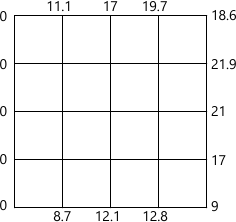MORE IN Engineering Mathematics 3
VTU Computer Science (Semester 3)
Engineering Mathematics 3
December 2011
Total marks: --
Total time: --
INSTRUCTIONS
(1) Assume appropriate data and state your reasons
(2) Marks are given to the right of every question
(3) Draw neat diagrams wherever necessary

1 (a) Obtain the Fourier series for the function$f(x)=\left\{\begin{matrix} -\pi x&; 0\le x \le 1 \\\pi (2-x) &;1\le x\le 2 \end{matrix}\right. ]\ and deduce that \[ \dfrac {\pi^2}{8}=\sum^\infty_{n=1}\dfrac {1}{(2n-1)^2}$
7 M
1 (b) Obtain the half range Fourier sine for the function. $f(x)=\begin{Bmatrix}1/4-x &;0 7 M 1 (c) Compute the constant term and the first two harmonics in the Fourier series of f(x) given by the following table.  x 0 1 2 3 4 5 f(x) 4 8 15 7 6 2 6 M 2 (a) Find the fourier transform of \[ f(x)= \left\{\begin{matrix} 1-x^2&for &|x|\le 1 \\0 &for &|x| >1 \end{matrix}\right.$ and hence evaluate $\int^\infty_0 \left ( \dfrac {x \cos x - \sin x}{x^3} \right )\cos \dfrac {x}{2}dx$
7 M
2 (b) Find the Fourier cosine transform of $f(x)=\dfrac {1}{1+x^2}$
7 M
2 (c) Solve the integral equation $\int^{\infty}_0d(\theta)\cos \alpha \theta d\theta=\left\{\begin{matrix} 1-\alpha&;&0 \le \alpha \le 1 \\0 &; & a>1 \end{matrix}\right. \ hence \ evaluate \ \int^{\infty}_0 \dfrac {\sin^2 t}{t^2}dt.$
6 M

3 (a) Solve two dimesional Laplace equation uxx+uyy=0, by the method of separation of variables.
7 M
3 (b) Solve the one dimensional heat equations $\dfrac {\partial u}{\partial t}=\dfrac {c^2\partial^2 u}{\partial x^2}, 0 (i) u(0,+)=0,u(?,t)=0 (ii) u(x,0)=u0 sinx where u0 = constant ± 0. 7 M 3 (c) Obtain the D' Almbert's solution of one dimensional wave equation. 6 M 4 (a) Fit a curve of the form y=aebx to the following data:  x: 77 100 185 239 285 y: 2.4 3.4 7 11.1 19.6 7 M 4 (b) Using graphical method solve the L.P.P minimize z=20x1=10x2 subject to the constraints x1+2x2?40; 3x1+x2?0; 4x1+3x2? 60; x1?0; x2?0 6 M 4 (c) Solve the following L.P.P miximize z=2x1 + 3x2 + x3, subject to the constraints x1+2x2+5x3?19, 3x1+x2+4x3?25, x1?0, x2?0, x3?0 using simplex method. 7 M 5 (a) Using the Regula - falsi method, find the root of the equation xex =cosx that lies between 0.4 and 0.6 Carry out four interations. 7 M 5 (b) Using relaxation method solve the equations. 10x-2y-3z=205; -2x+10y-2z=154; -2x-y+10z=120 7 M 5 (c) Using the Rayleigh's power method, find the dominant eigen value and the corresponding eigen ector of the matrix \[ A=\begin{bmatrix} 6&-2 &2 \\ -2&3 &-1 \\2 &-1 &3 \end{bmatrix}$ starting with the initial vector [1, 1, 1]T
6 M

6 (a) From the following table, estimate the number of students who have obtained the marks between 40 and 45:
 Marks 30-40 40-50 50-60 60-70 70-80 Number of student 31 42 51 35 31
7 M
6 (b) Using Lagrange's formula, find the interpolating polynomial that approximate the function described by following table:
 x 0 1 2 5 f(x) 2 3 12 147

Hence find f(3).
7 M
6 (c) A curve is drawn to pass through the points given by the following table:
 x 1 1.5 2 2.5 3 3.5 4 y 2 2.4 2.7 2.8 3 2.6 2.1

Using Weddle's rule, estimate the area bonded bt the curve, the x-axis and the lines x=1, x=4.
6 M

7 (a) Solve the Laplace's equation uxx+uyy=0, given that;7 M
7 (b) $Solve \ \dfrac {\partial^2u}{\partial t^2}=4 \dfrac {\partial^2 u}{\partial x^2}$ subject to u(0,t)=0; u(4,t)=0; u(x,0)=x (4-x). Take h=1, k=0.5
7 M
7 (c) Solve the equation $\dfrac {\partial u}{\partial t}=\dfrac {\partial^2 u}{\partial x^2}$ subject to the conditions u(x,0)=sinx, 0?x?1; u(0, t)=u(1, t)=0 using Schmidt's method. Carry out computations for two levels, taking h-1/3, k=1/36.
6 M

8 (a) Find the Z-transform of : $i) \ (2n-1)^2 \\ ii) \ \cos \left (\dfrac {n\pi}{2}+\pi/4 \right )$
7 M
8 (b) Obtain the inverse Z-transform of $\dfrac {4x^2-2z}{z^3-5z^2+8z-4}$
7 M
8 (c) Solve the difference equation yn+2 +6yn+1+9yn=2n with y0=y1=0 using Z transforms.
6 M

More question papers from Engineering Mathematics 3